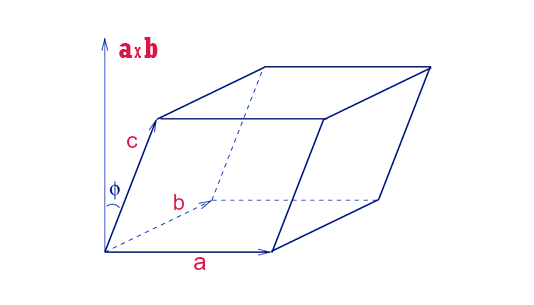# Volume of Parallelepiped Formula

In geometry, a parallelepiped is a three-dimensional figure formed by six parallelograms. Any of the three pairs of parallel faces can be viewed as the base planes of the prism. The volume of the parallelepiped can be found if the area of the bottom and height is known. The standard notation of the parallelepiped volume is V.Volume (V) = abc

### Formula of Volume of Parallelepiped

Let S is the area of the bottom and h is the height of a parallelepiped, then the volume formula is,

$\large V=S\times h$

Where,
S = Area of the bottom
h = Height

### Volume of Parallelepiped Formula Solved Example

Question: Find the volume of the parallelepiped, when

$$\begin{array}{l}20\,cm^{2}\end{array}$$
is the area of the bottom and 10 cm is the height of the parallelepiped.

Solution:

Given,
Aare of the botton = S =

$$\begin{array}{l}20\,cm^{2}\end{array}$$

Height = h = 10 cm

Volume formula is:

$$\begin{array}{l}\large V = S\times h\end{array}$$

$$\begin{array}{l}V=20\times10\end{array}$$

$$\begin{array}{l}V=200\,cm^{3}\end{array}$$